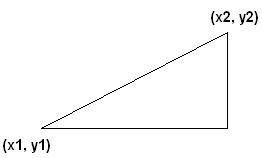# 1. Maths Distance Formula

The distance formula, like pythagoras theorem, can be used to determine collision detection quite easily.

First off, you must know the points at which the objects are located.

Say object 1 is located at (x1, y1) and object 2 is located at (x2, y2). Both on a 2 dimensional plane.Now I must clarify here that sqrt means the square root. There is no square root key on my keyboard, and in most programming languages it is called sqrt or sqr.

Ok now the distance formula is:

D = sqrt( (x2 – x1)^2 + (y2 – y1) ^2)

Which expanded looks like:

D = sqrt( (x2 – x1)*(x2 – x1) + (y2 – y1) *(y2 – y1))

By using this, if D is either 0 or less than a set collision distance we need, then there is a collision.

But how would we do this in a 3D world you might be wondering.

Well don’t wonder any longer, it is almost the same. You take your points which are located at:
(x1, y1) and (x2, y2)

Then simply add in the z values. So they would be located at:

(x1, y1, z1) and (x2, y2, z2)

And to get this into our equation, we add the z2 value take the z1 value and square it.

So our formulae now looks like:

D = sqrt( (x2 – x1)^2 + (y2 – y1) ^2 + (z2 – z1) ^2)

Which when expanded looks like:

D = sqrt( (x2 – x1)*(x2 – x1) + (y2 – y1) *(y2 – y1) + (z2 – z1) *(z2 – z1))

And there we have it, we can calculate the distance between 2 points in either 2d or 3d space.

If you have any questions, please email me at swiftless@gmail.com

• March 25, 2010